## Linearly Dependent VectorsVectors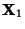,, ...,are linearly dependent Iff there exist Scalars,, ...,, not all zero, such that(1)

where Einstein Summation is used and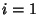, ...,. If no such Scalars exist, then the vectors are said to be linearly independent. In order to satisfy the Criterion for linear dependence,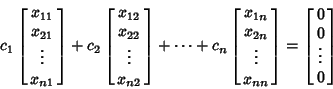(2)(3)

In order for this Matrix equation to have a nontrivial solution, the Determinant must be 0, so the Vectors are linearly dependent if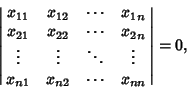(4)

and linearly independent otherwise.

Letandbe-D Vectors. Then the following three conditions are equivalent (Gray 1993).

1.andare linearly dependent.

2..

3. The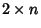Matrixhas rank less than two.

References

Gray, A. Modern Differential Geometry of Curves and Surfaces. Boca Raton, FL: CRC Press, pp. 186-187, 1993.